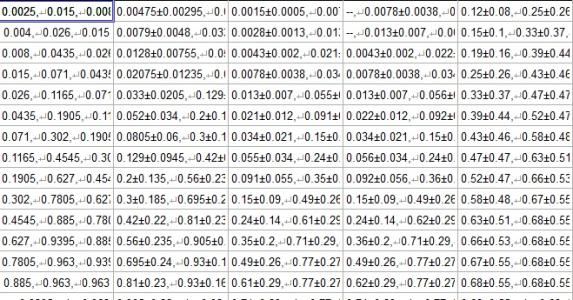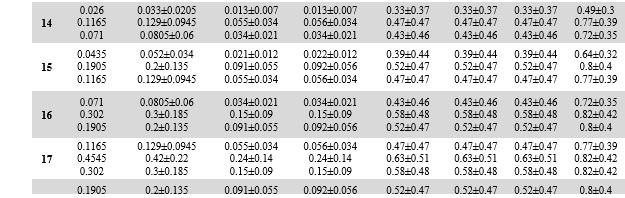# Merging tables and entering merged values in individual lines within a cellHi,

I am merging 3 tables together. I need the cells of the merged table to come out in a certain format. So, let's assume that first cell of tables 1, 2 and 3 has values a, b and c respectively. My IGOR program merges the tables to give me "a,b,c" as the first cell of the merged table. However, now I want each value to be reported in a new line within the cell. So instead of a,b,c  , I am looking for:

a

b

c

To do this, I tried adding "\r" ahead of b and c in my program but it hasn't helped. Attached is an image of the current output table, and also attached is an image of how I want the data to look. Current table output has the line change symbol ahead of b and c within each cell, but when I copy this table over to excel, the line change is not executed. Ultimately, I want to move this table to a word file. Kindly advise how to make this happen.

Thanks a lot,

PeeyushI don't understand the approach. How do the initial "3 tables" look like? Are these 3 waves (text or numeric)?

If you want to produce formatted output for some sort of document, do you need to generate it from the data format shown in your first figure, or can start from the original form whatever that is? Your problem seems to be related to the first column of the second figure (14,15,16 ...). I'd ignore that in Igor and just do the other columns here. I have a fairly strong aversion against Excel, but I guess the final step could easily be done there.

If the formatted table can be inserted as pdf in the Word file, maybe these snippets might be useful and a starting point for your own needs:

https://www.wavemetrics.com/code-snippet/wave2latex

Hi ChrLie,

The intial three tables are all 2D matrices (23x12) with one value in each cell. So basically normal tables. I am working with text 2D waves but numeric tables would also work using the num2str function while merging.

I am executing (with loop over 23 rows and 12 columns):

Mergedtable[i][j]=table1[i][j]+","+table2[i][j]+","+table3[i][j]    (when each original table is text 2D wave)

This gives me an output per cell as x,y,z.

However, instead of horizontal ouput like x,y,z,  I need output with line breaks as shown in the second attached figure.

The first column of the second figure (14,15,16..) was manually inserted into the table later.

Thanks for sharing the link. I will check it out!

Sincerely,

Peeyush

So, as I see it, your most pressing problem is not that Igor Pro does not create the table that you want. Your most pressing problem is that you cannot get the table from Igor Pro into a spreadsheet in Excel *while maintaining the \r as the recognized carriage return*. You suggest that you also cannot do the translation / import into Word (although perhaps you have not tried directly, I cannot tell).

If this is the real gist of your problem, you are (a whole heck of a lot) out of luck. Going directly between tables in Igor Pro and spreadsheets in Excel can be tricky even in the best cases. You add formatting controls in the cells that manage to make the translation even more difficult.

I suggest that you consider other approaches:

* Export the data directly to a CSV or tab-delimited file. Import to Excel. Play around with the macro-programming in Excel to get what you want.

* Write the data to a Notebook in Igor Pro (rather than to a text-based set of waves). Export the Notebook file. Pull the Notebook file into Word. Play around with the formatting styles in Word to get what you want.

* Export the data directly to a set of CSV files. Develop an import method in LaTeX using the datatool package to create the table format that you want using the CSV files as the databases. <-- Given the complexity of the table that you seem to want to create, this would likely be my approach.

Hi jjweimer,

Thanks a lot for these suggestions! I'll try to work with these. Meanwhile, here is one thing I had done which worked but with an issue:

In my IGOR program which merges three tables into one,

Instead of executing this: (from my comment above)

Mergedtable[i][j]=table1[i][j]+","+table2[i][j]+","+table3[i][j],

I executed a modified version of this line which created output in each cell of the merged table that looked like this:

"=value1&CHAR(10)&value2&CHAR(10)&value3"   (quotes excluded)

where value 1, value 2 and value 3 are values from the original 3 tables which I merged.

Now, &CHAR(10)& is line break function in excel. So when I simply copied this merged table into excel and clicked "wrap text". It worked!

BUT, I ran into a slight problem still which is that I wanted all numbers to appear in scientific notation i.e. 0.00E+00 format. But in excel, I didn't get scientific notation in my merged table. Instead it gave me regular numbers.

So new question: Is there a way to fix the scientific notation format in my 3 original text 2D tables so that after merging when I move them to excel, it doesn't convert them to general numeric notation while text wrapping?

Sincerely,

Peeyush

I'm still not entirely sure what your goal is but if you want to merge and rearrange the order, does this what you want to achieve?:

// make some dummy waves
make/O/T/n=(32,12) txt1, txt2, txt3
txt1 =num2str(1)
txt2 =num2str(2)
txt3 =num2str(3)

// make output wave
make/t/n=(3*32,12) mrgd

// alternate rows of txt waves in output
mrgd[0, ;3][] = txt1[trunc(p/3)][q]
mrgd[1, ;3][] = txt2[trunc(p/3)][q]
mrgd[2, ;3][] = txt3[trunc(p/3)][q]

As a side note:

Mergedtable[i][j]=table1[i][j]+","+table2[i][j]+","+table3[i][j]

This can be written without a loop as

Mergedtable[][]=table1[p][q]+","+table2[p][q]+","+table3[p][q]